﻿ 怒江电网黑启动网架重构阶段最优路径研究

# 怒江电网黑启动网架重构阶段最优路径研究Research on Optimal Path of Black Start Grid Reconfiguration Stage of Nujiang Power Grid

Abstract: In this paper, Dijkstra algorithm is used to establish the target network for path optimization in the grid reconstruction stage during the black start process of Nujiang Power Grid. The Nujiang Power Grid is divided into four black start subsystems in the Lushui area, the Gongshan area, the Fugong area and the Lanping area, and the target grids are respectively established. Taking the power grid switch station, network hub substation and hydropower station as the nodes in the black start process, the connection line between the switch stations, network hub substations and hydropower stations in each area is taken as the side. Considering the important loads and branch parameters in the black-start process, the weighting values of each edge are given, and finally find the shortest path from the specified starting node to the end point and mark the shortest path. The Dijkstra algorithm is used to find the optimal path for the black start network reconstruction and reduce the black start time. The main task of the grid reconstruction phase is to send power to the power plant and establish a stable grid structure, and restore the load for the next stage. It is important to lay a solid foundation for the whole process of black start.

1. 引言

2. 网架重构

2.1. 恢复路径的选择

1) 恢复路径中电压转换的次数要尽量少。由于启动电源和待恢复目标在网络中的具体位置的不同，恢复路径往往会涉及到电压等级的多次转换。

2) 恢复路径的长度要尽量短。

3) 恢复路径经过节点的拓扑重要性尽可能高。节点的重要度描述了节点在网络拓扑结构中的位置和作用。

4) 恢复路径上的被启动电源或负荷的优先级应尽可能高。使恢复路径首先辐射到系统中重要性等级较高的机组和负荷，以有利于这些机组与负荷的快速恢复。

5) 恢复路径一次设备的操作次数应尽量减少。

6) 恢复路径应具备技术可行性。在黑启动初期，技术可行性主要通过数值仿真计算，检验发电机自励磁，线路空载合闸过电压，电压和频率稳定性及小系统低频振荡等项目是否符合运行安全要求。

2.2. 网架重构的数学模型

2.2.1. 目标函数

$\mathrm{max}f=\underset{i=1}{\overset{m}{\sum }}\alpha {x}_{i}{L}_{imi}+\underset{j=1}{\overset{m}{\sum }}\beta {x}_{j}{L}_{gej}$ (1)

2.2.2. 约束条件

1) 潮流约束

$\left\{\begin{array}{c}{P}_{i}={V}_{i}{\sum }_{j=1}^{M}{V}_{j}\left({G}_{ij}\mathrm{cos}{\delta }_{ij}+{B}_{ij}\mathrm{sin}{\delta }_{ij}\right)=0\\ {Q}_{i}={V}_{i}{\sum }_{j=1}^{M}{V}_{j}\left({G}_{ij}\mathrm{cos}{\delta }_{ij}-{B}_{ij}\mathrm{sin}{\delta }_{ij}\right)=0\end{array}$ (2)

2) 发电机节点功率约束

$\left\{\begin{array}{c}{P}_{Gi}^{\mathrm{min}}\le {P}_{Gi}\le {P}_{Gi}^{\mathrm{max}}\\ {Q}_{Gi}^{\mathrm{min}}\le {Q}_{Gi}\le {Q}_{Gi}^{\mathrm{max}}\end{array}$ (3)

3) 线路传输功率极限约束

${P}_{Li}\le {P}_{Li\mathrm{max}}$ (4)

2.3. 确定路径权值

3. Dijkstra算法

Dijkstra (迪杰斯特拉)算法是一种单源最短路径算法，即在图中求出给定顶点到其它任一顶点的最短路径。运算的主要过程是以给定顶点为中心向外搜寻，直到找到所有的最短路径为止  。Dijkstra算法作为代表性的最短路径算法，被广泛介绍在如图论、运筹学、算法导论等各种专业课中。这种算法的限制是不允许图中存在负权边  。

3.1. 算法原理

(1) 初始时，S只包含源点，即 $S=\left\{v\right\}$，v到v的距离为0。其他所有顶点都在集合U中。在源点与集合U中的顶点邻接时距离 $〈u,v〉$ 有正常权值，反之则距离 $〈u,v〉$ 权值为正无穷。

(2) 将集合U中的点加入集合S中，选取的点k必须与源点v邻接且保证距离最短。

(3) 对源点v到集合U中各顶点的距离进行更新，以新加入集合S中的点k为中介点，重新搜索最短路径，若经过中介点k的最短路径距离相比之前变短，则以该距离取代之前的距离。

(4) 重复步骤(2) (3)，当集合U中没有点时，搜索完成。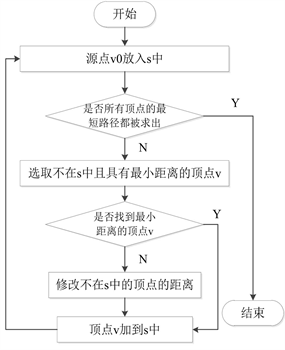Figure 1. Dijkstra algorithm flow chart

3.2. 基于网架结构的Dijkstra算法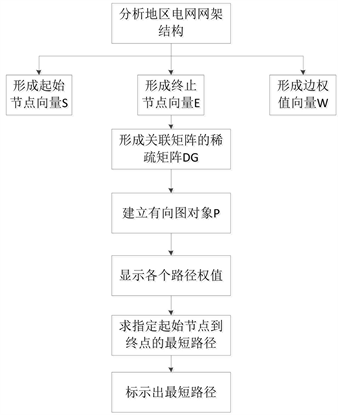Figure 2. Flow chart of Dijkstra algorithm based on grid structure

(1) 以黑启动过程中各地区电网开关站、网络枢纽变电站以及水电站为节点，以各地区开关站、网络枢纽变电站以及水电站间连接线路为边，综合考虑黑启动过程中重要负荷，支路参数等情况，给每一条边赋权值，形成起始节点向量S、终止节点向量E以及边权值向量W。

(2) 在步骤一分析地区电网形成起始节点向量、终止节点向量以及边权值向量的基础上构建关联稀疏矩阵DG。

(3) 建立地区电网有向图对象P并显示地区电网各路径权值。

(4) 求地区电网指定起始节点到终点的最短路径，即起始节点到终点的权值最小。求出指定节点间的最短距离、标出最短距离经过的路径节点。

(5) 标示出所指定的起始节点到目标节点的最短路径。

4. 算例分析

4.1. 泸水区域

4.2. 福贡区域Table 1. Summary of the results of each edge of the Lushui areaTable 2. Recovery path scheme comparison of the Lushui area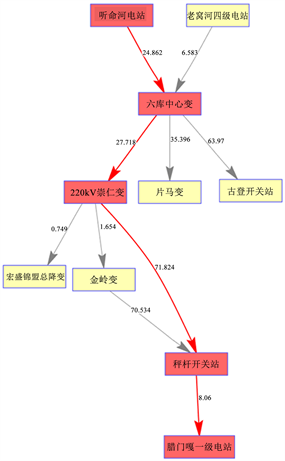Figure 3. Schematic diagram of optimal path reconstruction in the LushuiTable 3. Summary of the results of each edge of the Fugong area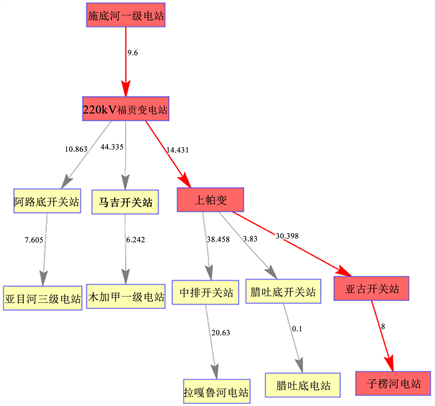Figure 4. Schematic diagram of optimal path reconstruction in the Fugong

4.3. 贡山区域Table 4. Summary of the results of each edge of the Gongshan area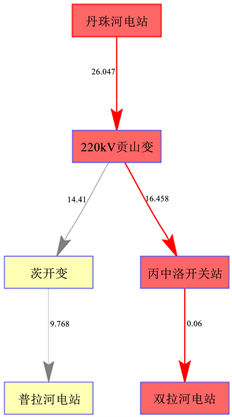Figure 5. Schematic diagram of optimal path reconstruction in the Gongshan

4.4. 兰坪区域Table 5. Summary of the results of each edge of the Lanping area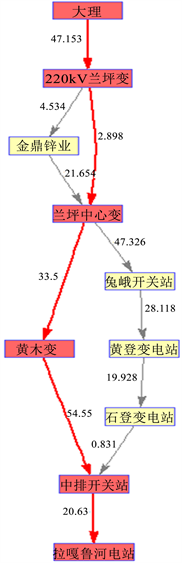Figure 6. Schematic diagram of optimal path reconstruction in the LanpingTable 6. Recovery path scheme comparison of the Lanping area

5. 结论

NOTES

*通讯作者。

 戴小俊. 电力系统黑启动研究[J]. 中国电力教育, 2012(33): 148-149.

 周云海, 刘映尚, 胡翔勇. 大停电事故后的系统网架恢复[J]. 中国电机工程学报, 2008, 28(10): 32-36.

 刘艳. 电力系统黑启动恢复及其决策支持技术的研究[D]: [博士学位论文]. 保定: 华北电力大学, 2007.

 顾雪平, 李少岩, 李凯. 用于网架重构决策的节点重要度动态评价方法[J]. 电力自动化设备, 2017, 37(4): 89-95.

 孙璞玉, 张焰, 罗雯清. 考虑网架覆盖率与分散度的网架重构优化[J]. 电网技术, 2015, 39(1): 271-278.

 Fink, L.H., Liou, K.L. and Liu, C.C. (1995) From Generic Restoration Actions to Specific Restoration Strategies. IEEE Transactions on Power Systems, 10, 745-752.
https://doi.org/10.1109/59.387912

 朱海南, 刘玉田. 考虑线路投入顺序的网架重构机组恢复多目标优化[J]. 电力系统自动化, 2014, 38(16): 53-59.

 刘连志, 顾雪平, 刘艳. 不同黑启动方案下电网重构效率的评估[J]. 电力系统自动化, 2009, 33(5): 24-28.

 林振智, 文福拴. 基于加权复杂网络模型的恢复路径优化方法[J]. 电力系统自动化, 2009, 33(6): 11-15, 103.

 Adibi, M.M. and Milanicz, D.P. (1999) Estimating Restoration Duration. IEEE Transactions on Power Systems, 14, 1493-1498.
https://doi.org/10.1109/59.801939

 刘强, 石立宝, 倪以信, 等. 电力系统恢复控制的网络重构智能优化策略[J]. 中国电机工程学报, 2009, 29(13): 8-15.

Top